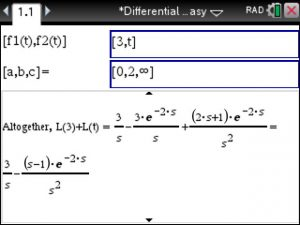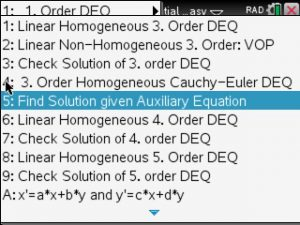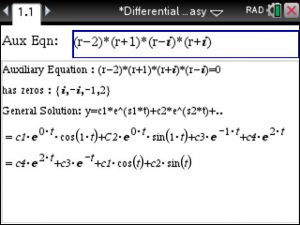## TiNspire CX CAS : Solving 1. order Differential Equations – Step by Step

If we are asked to solve the 1. Order Differential Equation y’+4y=8 we see that it is Linear in y and thus use option B in Differential Equations Made Easy at www.TiNspireApps.com as shown below:

Next enter the coefficients 4 and 8 and leave the 2. box empty since we are not given any initial conditions.

To get the general solution :

If we are given this first order homogeneous Differential Equation :

dy/dx + 5yx^3 = 0 we think of it as dy/dx = – 5yx^3 which calls for Separable Differential Equations which are solved using option 3 as follows :

Here we add an initial condition which allows to find a particular solution:

and finally the separation of variables method yields the particular solution:

## TiNspire CX : Euler Method (Differential Equations)

When solving a Differential Equation y’=y*(5-y) , y(0)=9 numerically using the Euler Method given stepsize of 0.1 use the Differential Equations made Easy app at www.tinspireapps.com and select Euler Method in the Menu as shown below :

Now you just enter the Differential Equation in the top box and the starting point and the step size in the bottom box as shown below:

The bottom box now shows the step by step solution of the Euler Method. Works correctly for any given Differential Equation.

Alternative to the Euler Method you may also the built-in Runga Kutta RK4 method.

## TiNspire CX CAS: Nonhomogeneous, Nonlinear Cauchy Euler 3. order Differential Equation

To solve a 3. order Cauchy Euler Differential that is Nonhomogeneous and NonLinear you would use the Differential Equations Made Easy at www.TiNspireApps.com and enter the coefficients of the Differential Equations as follows:

As can be seen, the substitution y=x^n allows us to find the zeros of the homogeneous Differential Equation and its solution below. Now, we are after the nonhomogenous solution which involves find the 4 Wronskians W, W1, W2, W3 using the Variation of Parameter method:

After finding the 3 v_i, their integration allows us to find the final solution

Puuh, that was a lot of work…If you want to skip watch all the steps you might just jump straight to the final solution.

## TiNspire CX CAS: Classify Nodes, Saddle Points in Stability of Non-Linear Systems

Say you have to classify nodes when studying the stability of Non-Linear Systems. Use the Differential Equations Made Easy App at www.TinspireApps.com under menu item 3 E . Enter the given System as follows in the two top boxes:

When plugging the critical points into the Jacobian we are able to compute the EigenValues that allow us to classify the nodes as stable, unstable, saddle point, center or spiral point.

and finally

Very Simple!

Posted on Categories differential equation, tinspirecx

## TiNSpire CX: Solve System of Differential Equations using LaPlace Transform – Step by Step

Say you have to solve the system of Differential Equations shown in below’s image. Launch the Differential Equations Made Easy app (download at www.TiNspireApps.com) , go to Laplace Transforms in the menu and just type in as shown below:

Scrolling down to view all steps finally shows the correct final answer:

Posted on Categories differential equation, laplace transform

## Solve Separable 1. Order Differential Equation using the TiNspire CX

To solve a separable Differential Equation such as dy/dx + xy=0 or rewritten dy/dx = – x*y with initial condition y(0)=2 use the Differential Equation Made Easy app at www.TinspireApps.com , use menu option 1 3 (Separation of Variables) and enter as follows :

to finally get both the general and particular solutions.

Posted on Categories differential equation

## Differential Gleichungen Loesen – Schrittweise – mit dem Ti-Nspire CX CAS

Nehmen wir als Bespiel die homogene Differentialgleichung 2. Ordnung :

y” + 8y’ + 16y =0

Wir starten die TiNspire APP “Differentialgleichungen Leicht Gemacht” von www.Tinspireapps.com und gehen im Menu zu Option 4: Homogene Differentialgleichung.

Und geben einfach die DGL oben ein.

Um eine partikulaere Loesung zu finden gibt man die Anfangswert Bedingungen unten ein:

So leicht ist es schrittweise Loesungen zu Differentialgleichungen zu bekommen. Man kann diese Loesung mit der von Symbol-Lab vergleichen unter : https://www.symbolab.com/solver/ordinary-differential-equation-calculator/y”%2B8y’%2B16y%3D0

Posted on

## TiNspire CX CAS : Solve Differential Equations – Step by Step – using LaPlace Transforms

Using the Differential Equations Made Easy APP at https://tinspireapps.com/?a=deqme you can solve Differential Equations using LaPlace Transforms as shown below:

Posted on Categories differential equation, laplace transform

## TiNspire: LaPlace Transforms of a Piecewise-Defined Function

Laplace transform over Piecewise def. Function

Example:

f(1) = 3 defined over 0<= t <2

f(2) = t  defined over t >= 2

To find the LaPlace Transform use Differential Equations Made Easy at

https://www.tinspireapps.com/?a=DEQME  and select LAPLACE TRANSFORM OF PIECEWISE DEFINED FUNCTION and enter as follows:We use infinity since the function f2 is not bounded. If it was bounded by for example 10 then we would have entered as [0,2,10]

Posted on Categories differential equation, laplace transform

## Auxiliary / Characteristic Equation to Solution of Differential Equation – Step by Step – using the TiNSpire CX

Auxiliary Equation Solution using the Tinspire CX

Finding the Solution given Auxiliary (also called Characteristic) Equation of a Differential Equation is a fun exercise using the Differential Equation Made Easy app .

Just select option 5 :Then enter the auxiliary equation (of any order)  as shown belowPosted on Categories differential equation